SEARCH HOMEMath Central Quandaries & QueriesQuestion from Bill, a teacher: A student asked me where did the "difference of cubes" and "sum of cubes" come from.  I did not have an answer for her. She is very bright and understands how they work but wanted to know where they derived from.  Any help you can offer would be great.  ThanksHi Bill.

A difference of cubes is of course a perfect cube minus a perfect cube.

I assume your student already has the formula

a3 - b3 = (a - b)(a2 + ab + b2)

We can prove this using polynomial division.

First, we look at the roots of a3 - b3 and immediately we can see that if a = b, then a3 - b3 = 0, so (a-b) is a factor.

Next, rewrite it: a3 - b3 = a3 + 0a2b + 0ab2 - b3 and use synthetic (or long) division to find the other factor. I'll use long division: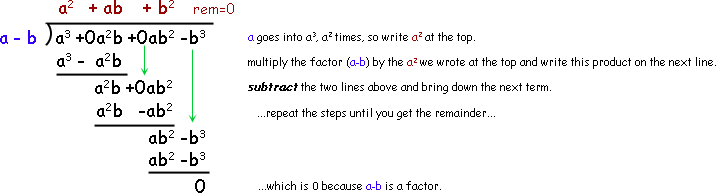You could do something very similar for a sum of cubes.

However, there is a geometric way of seeing this too, at least where a and b are positive and a > b:

For example, say a= 4 and b = 2. Here is 43 - 23: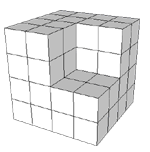and here is 33 - 23 :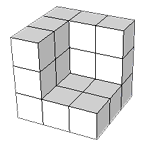Let's take apart 33 - 23 first. The red object is broken into the other colours: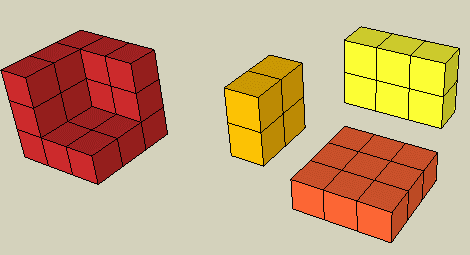So the red object is composed of the orange piece which is 32 plus the yellow piece which is 3x2 and the gold piece which is 22. So the original 33 - 13 equals the components 32 + 3x2 + 22. Now since (3-2) = 1, we can multiply by this and not change anything, so we've shown

33 - 23 = (3-2) (32 + 3x2 + 22)

But what about trying something harder, like 53 - 23?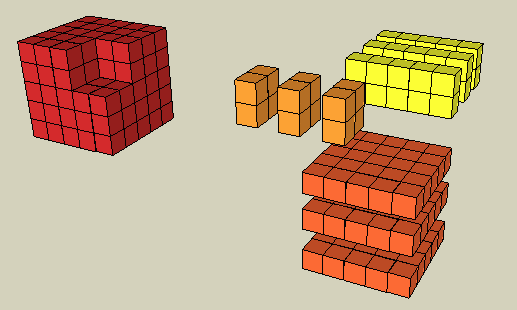We have the same breakdown, but there are 3 copies of everything! This makes sense because (5-2) = 3.

So we've shown that

53 - 23 = (5-2) (52 + 5x2 + 22)

just as the formula predicted and our long division proved algebraically.

If you look carefully at the last diagram, you can consider it as a3 - b3 and show that the number of copies is always (a-b), thus proving the general case.

I'd encourage your student to explore in this way the sum of cubes and see if she can come up with a similar algebraic and geometric solution.

Cheers,
Stephen La Rocque.Math Central is supported by the University of Regina and The Pacific Institute for the Mathematical Sciences.